# Regularity is product-closed

This article gives the statement, and possibly proof, of a topological space property satisfying a topological space metaproperty
View all topological space metaproperty satisfactions | View all topological space metaproperty dissatisfactions
|
Property "Page" (as page type) with input value "{{{property}}}" contains invalid characters or is incomplete and therefore can cause unexpected results during a query or annotation process.
Property "Page" (as page type) with input value "{{{metaproperty}}}" contains invalid characters or is incomplete and therefore can cause unexpected results during a query or annotation process.

## Statement

### Verbal statement

An arbitrary (finite or infinite) product of regular spaces, when endowed with the product topology, is also a regular space.

## Definitions used

### Regular space

Further information: Regular space

A topological space$X$ is regular if it is T1 and further, if given a point$x \in X$ and an open set$U$ containing$x$, there is an open set$V$ containing$x$ such that$\overline{V} \subset U$.

### Product topology

Further information: Product topology

Suppose$I$ is an indexing set, and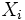$X_i$ a family of topological spaces,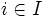$i \in I$. Then if$X$ is the Cartesian product of the$X_i$s, the product topology on$X$ is a topology with subbasis given by all the open cylinders: all sets of the form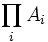$\prod_i A_i$ such that for all but one$i$,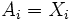$A_i = X_i$, and for the one exceptional$i$,$A_i$ is an open subset of$X_i$.

A basis for this topology is given by finite intersections of open cylinders: these are products where finitely many coordinates are proper open subsets, and the remaining are whole spaces.

## Related facts

An analogous proof to this one shows that the property of being a regular space is also closed under taking arbitrary box products. Note that this statement is independent of the statement about arbitrary products; neither can be deduced directly from the other, because the property of being regular is not closed either under passing to a coarser topology or under passing to a finer topology.

Further information: Regularity is box product-closed

## Proof

### Proof outline

The proof proceeds as follows:

• Start with the point in the product space, and the open set containing it
• Find a basis open set containing the point, which lies inside this open set
• For each coordinate on which the projection of the basis open set is a proper subset, find a smaller open subset whose closure is contained inside the given projection
• Reconstruct from these a smaller basis open set whose closure lies in the given basis open set

### Proof details

Given: Indexing set$I$, a family$\{ X_i \}_{i \in I}$ of regular spaces.$X$ is the product of the$X_i$s, given the product topology

To prove:$X$ is a regular space

Proof: It suffices to show that given any point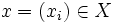$x = (x_i) \in X$, and any open subset$U$ of$X$ containing$x$, there is an open subset$V \ni x$ such that$\overline{V} \subset U$.

First, there exists a basis element of$X$ containing$x$, and inside$U$. Suppose this basis element is taken as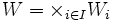$W = \times_{i \in I} W_i$. Now, for those$W_i$ that are equal to the whole space, define$V_i = W_i$. For those$W_i$ that are proper open subsets of$X_i$, use the fact that$X_i$ is regular to find an open set$V_i \ni x_i$ in$X_i$, such that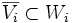$\overline{V_i} \subset W_i$.

Now consider$V$ to be the product of all the$V_i$s. Clearly$x \in V$ by construction, and$V$ is a basis element for the topology on$X$. In particular,$V$ is open. Further, the closure of$V$ is contained in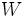$W$, and hence in$U$. Thus,$V$ is as we sought, and the proof is complete.